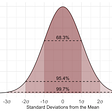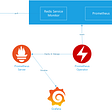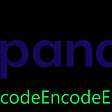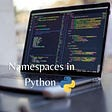# Fast loading and saving of `pandas `data frames using `numpickle` (`numpy `and `pickle`)

Loading huge data frames (Giga bytes size) from text files (csv or tsv) can be pretty time consuming.

Here, I show you how you can save 3x — if not several magnitudes of order — time by using the `numpy` and `pickle` packages for saving and loading`pandas` data frames.

If the data frame consists of numeric values-only without any non-numeric values (e.g. strings), the loading is in average ~7x faster.

In the following, you see the code:

`import pandas as pdimport numpy as npimport pickleimport osdef save_numpickle(df, outfpath, all_numeric=False):    arr, colnames, rownames = df.to_numpy(), df.columns, df.index    np.save(arr=arr, file=outfpath)    pickle.dump({‘colnames’: colnames,                  ‘rownames’: rownames,                  ‘dtypes’: None if all_numeric else df.dtypes},                 open(outfpath + “.pckl”, “wb”))def load_numpickle(fpath):    df = pd.DataFrame(np.load(fpath, allow_pickle=True))    with open(fpath + “.pckl”, “rb”) as fin:        meta = pickle.load(fin)    # if no ‘types’ present, assuming all_numeric    df.index, df.columns, dtypes = \        meta[‘rownames’], meta[‘colnames’], meta.get(‘dtypes’, None)    if dtypes is not None:        df = df.astype(dtypes)    return df`

The code is made accessible for you from everywhere:

`pip install numpickle`

`import pandas as pdimport numpickle as npl# create example data frame with non-numeric and numeric columnsdf = pd.DataFrame([[1, 2,'a'], [3, 4, 'b']])df.columns = ["A", "B", "C"]df.index = ["row1", "row2"]df#       A  B  C# row1  1  2  a# row2  3  4  bdf.dtypes# A     int64# B     int64# C    object# dtype: object# save data frame as numpy array and pickle row and column names# into a helper pickle file "/home/user/test.npy.pckl"npl.save_numpickle(df, "/home/user/test.npy")# load the saved data as a control:df_ = npl.load_numpickle("/home/user/test.npy")df_#       A  B  C# row1  1  2  a# row2  3  4  bdf_.dtypes# A     int64# B     int64# C    object# dtype: objectall(df == df_)# True`

In case you have numeric-only values, set `all_numeric=True` (This accelerates the loading ~7x):

`df = pd.DataFrame([[1, 2], [3, 4]])df.columns = ["A", "B"]df.index = ["row1", "row2"]# save the data framenpl.save_numpickle(df, "/home/user/test_num.npy", all_numeric=True)# load the saved datadf_ = npl.load_numpickle("/home/user/test_num.npy")`

## Preprocess your data file for fast loading by `numpickle`

How to convert any tabular text file to a fast-loadable`numpickle` file:

`def save_file_as_numpickle(fpath,                            sep=”\t”,                            ending=".tsv",                            all_numeric=False,                            deletep=False,                            *args, **kwargs):    df = pd.read_csv(fpath, sep=sep, *args, **kwargs)    save_numpickle(df, fpath.replace(ending, ".npy"),                        all_numeric=all_numeric)    if deletep:        os.remove(fpath)# usage:fpath = /path/to/input.csvimport numpickle as nplnpl.save_file_as_numpickle(fpath,                            sep=",", # comma separated file!                           ending=".csv", # ending to be replaced by ".npy"                           all_numeric=True,                           deletep=True)# now, you can do:df = npl.load_numpickle(fpath.replace(".csv", ".npy"))# you should be able to feel the difference in loading speed!`

--

--

--

## More from Gwang Jin Kim

Code tweaker + Human Geneticist (Ph.D.) | Join medium by: https://gwang-jin-kim.medium.com/membership

Love podcasts or audiobooks? Learn on the go with our new app.

## List Comprehension In Python## Frictionless Validity Checks## Statistical / Statistic Process Control (SPC) for Laymen## HyperDao - a DAO based IDO launchpad Coming Soon❗️🔥 Offering Unparalleled: - Transparency ⭐️ …## Setting Up Dynamodb For Local Development## Deploying and monitoring a Redis cluster to Oracle Container Engine (OKE)## Introducing Conjure, Palantir’s toolchain for HTTP/JSON APIs## Pulse Sensor for Arduino## Gwang Jin Kim

Code tweaker + Human Geneticist (Ph.D.) | Join medium by: https://gwang-jin-kim.medium.com/membership

## Taming larger-than-RAM-data workflows: a Dask example## How to get around of the “UnicodeEncodeError: ‘utf-8’ codec can’t encode character …: surrogates…## Installing Anaconda for Python Development## Namespaces in Python